### Home > CCA > Chapter Ch8 > Lesson 8.1.2 > Problem8-17

8-17.
1. Use the process you developed in problem 8-14 to factor the following quadratics, if possible. Homework Help ✎

1. x2 − 4x − 12

2. 4x2 + 4x + 1

3. 2x2 − 9x − 5

4. 3x2 + 10x − 8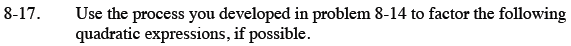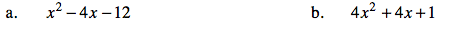Use a generic rectangle and a diamond to factor the quadratic.

(x − 6) (x + 2)

See the hints in part (a).

(2x + 1)2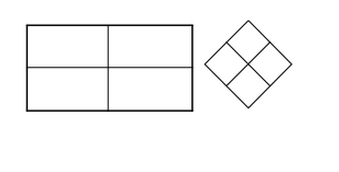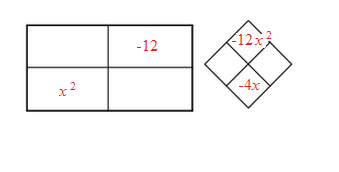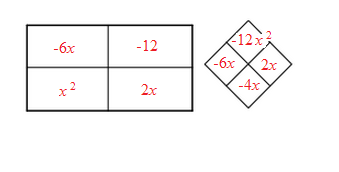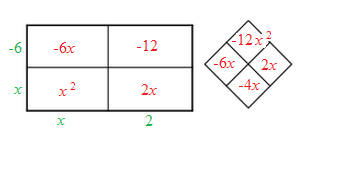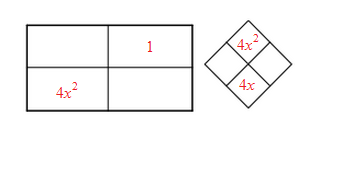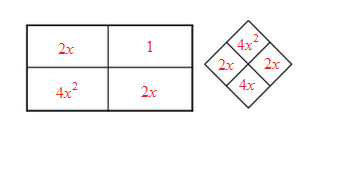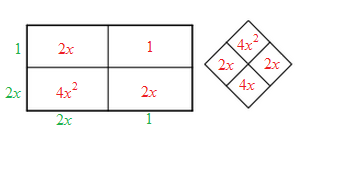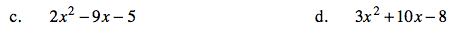See the hints in part (a).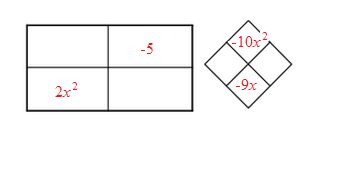See the hints in part (a).